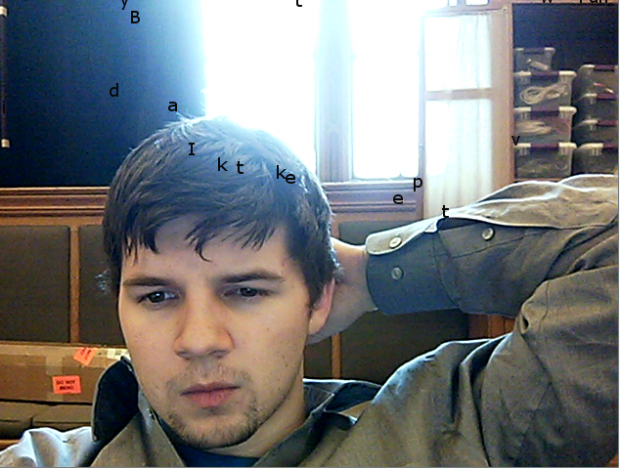# Andy

## 28 Jan 2013```
import processing.video.*;

Capture cam;
PFont f;
PImage bg;
final int WIDTH = 640;
final int DARK_THRESHOLD = 120;
Letter[] fallers;
Letter[] droppers;
int dropCount = 0;
final String[] WORDS = {"H","i","m","y","n","a","m","e",
"i","s","A","n","d","y","B","i",
"a","r","a","d","I","l","i","k",
"e","t","o","m","a","k","e","t",
"h","i","n","g","s","f","o","r",
"p","e","o","p","l","e","t","o",
"d","i","s","c","o","v","e","r",
"w","i","t","h","f","u","n","!"};

void setup() {
size(WIDTH, 480);

String[] cameras = Capture.list();
if (cameras.length == 0) println("No cameras");
else cam = new Capture(TextRain.this, cameras);
cam.start();

f = createFont("Verdana", 18, true);
textFont(f);
fill(10);

fallers = new Letter[WIDTH/10];
droppers = new Letter[WIDTH/10];
}

int nextDrop() {
if (dropCount >= WIDTH/10) return -1;

int rand = int(random(WIDTH/10));
while(fallers[rand] != null) {
rand = (rand + 1) % (WIDTH/10);
}

return rand;
}

void draw() {
// Get background from webcam
image(cam, 0, 0);

// Drop a letter
if (dropCount < 64 && (millis() / 1000) > dropCount) {
int nextDrop = nextDrop();
if (nextDrop != -1) {
fallers[nextDrop] = new Letter(WORDS[nextDrop%WORDS.length],
nextDrop*10, 0);
}
dropCount++;
}

// Draw letters
for (int i = 0; i < fallers.length; i++) {
Letter n = fallers[i];
if (n != null) text(n.letter, n.x, n.y);
}

// Gravity
for (int i = 0; i < fallers.length; i++) {
if (fallers[i] != null) {
Letter a = fallers[i];
if (a.y >= height || a.y < 0) continue;
color c = pixels[WIDTH * a.y + a.x];
float r, g, b;
r = red(c); g = green(c); b = blue(c);
int brightness = int(.2989*r + .5870*g + .1140*b);
if (brightness > DARK_THRESHOLD) fallers[i].y += 1;
}
}

// Reset falling letters
for(int i = 0; i < fallers.length; i++) {
if (fallers[i] != null && fallers[i].y >= height) {
fallers[i].y = 0;
}
}
}

public class Letter {
public String letter;
public int x;
public int y;

public Letter(String letter, int x, int y) {
this.letter = letter;
this.x = x;
this.y = y;
}
}

```

I think Text Rain is one of my weaker projects in the upkit intensive, but here it is. I used an object-oriented approach, creating a Letter class which stored it’s x and y position as well as the character to be drawn. Other than that, my implementation is likely pretty vanilla – I used the Capture object to get pixels from the webcam, loaded them into an array, and read points under the letters for darkness above a certain threshold. Definitely a fun starter project! Also, this is the first time in my life I have ever intentionally not shaved my stache, and I think it looks pretty good in this photo: